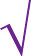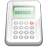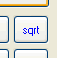# Squares and Square Roots

First learn about Squares, then Square Roots are easy.

## How to Square A Number

To square a number: multiply it by itself.

### Example: What is 3 squared?

 3 Squared == 3 × 3 = 9

"Squared" is often written as a little 2 like this:This says "4 Squared equals 16"
(the little 2 says the number appears twice in multiplying)

## Squares From 02 to 62

 0 Squared = 02 = 0 × 0 = 0 1 Squared = 12 = 1 × 1 = 1 2 Squared = 22 = 2 × 2 = 4 3 Squared = 32 = 3 × 3 = 9 4 Squared = 42 = 4 × 4 = 16 5 Squared = 52 = 5 × 5 = 25 6 Squared = 62 = 6 × 6 = 36

 The squares are also on the Multiplication Table:## Negative Numbers

We can also square negative numbers.

### Example: What happens when we square (−5) ?

(−5) × (−5) = 25

(because a negative times a negative gives a positive)

That was interesting!

When we square a negative number we get a positive result.

Just the same as squaring a positive number:(For more detail read Squares and Square Roots in Algebra)

## Square Roots

A square root goes the other way:3 squared is 9, so a square root of 9 is 3

A square root of a number is ...

... a value that can be multiplied by itself to give the original number.

A square root of 9 is ...

... 3, because when 3 is multiplied by itself we get 9.

What can we multiply by itself to get this?To help you remember think of the root of a tree: "I know the tree, but what root made it?" In this case the tree is "9", and the root is "3".

Here are some more squares and square roots:4 16 5 25 6 36 7 49

## Decimal Numbers

It also works for decimal numbers.

Try the sliders below (note: '...' means the decimals continue on forever):

Using the sliders:

• What is the square root of 8?
• What is the square root of 9?
• What is the square root of 10?
• What is 1 squared?
• What is 1.1 squared?
• What is 2.6 squared?

## Negatives

We discovered earlier that we can square negative numbers:

### Example: (−3) squared

(−3) × (−3) = 9

And of course 3 × 3 = 9 also.

So the square root of 9 could be −3 or +3

### Example: What are the square roots of 25?

(−5) × (−5) = 25

5 × 5 = 25

So the square roots of 25 are −5 and +5

## The Square Root SymbolThis is the special symbol that means "square root", it is sort of like a tick, and actually started hundreds of years ago as a dot with a flick upwards. It is called the radical, and always makes mathematics look important!

We use it like this:and we say "square root of 9 equals 3"

### Example: What is √25?

25 = 5 × 5, in other words when we multiply 5 by itself (5 × 5) we get 25

√25 = 5

But wait a minute! Can't the square root also be −5? Because (−5) × (−5) = 25 too.

• Well the square root of 25 could be −5 or +5.
• But when we use the radical symbol we only give the positive (or zero) result.

### Example: What is √36 ?

Answer: 6 × 6 = 36, so √36 = 6

## Perfect Squares

The Perfect Squares (also called "Square Numbers") are the squares of the integers:

 Perfect Squares 0 0 1 1 2 4 3 9 4 16 5 25 6 36 7 49 8 64 9 81 10 100 11 121 12 144 13 169 14 196 15 225 etc...

Try to remember them up to 12.

## Calculating Square Roots

It is easy to work out the square root of a perfect square, but it is really hard to work out other square roots.

### Example: what is √10?

Well, 3 × 3 = 9 and 4 × 4 = 16, so we can guess the answer is between 3 and 4.

• Let's try 3.5: 3.5 × 3.5 = 12.25
• Let's try 3.2: 3.2 × 3.2 = 10.24
• Let's try 3.1: 3.1 × 3.1 = 9.61
• ...

Getting closer to 10, but it will take a long time to get a good answer!At this point, I get out my calculator and it says: 3.1622776601683793319988935444327 But the digits just go on and on, without any pattern. So even the calculator's answer is only an approximation !

Note: numbers like that are called Irrational Numbers, if you want to know more.

## The Easiest Way to Calculate a Square RootUse your calculator's square root button!And also use your common sense to make sure you have the right answer.

## A Fun Way to Calculate a Square Root

There is a fun method for calculating a square root that gets more and more accurate each time around:

 a) start with a guess (let's guess 4 is the square root of 10)b) divide by the guess (10/4 = 2.5) c) add that to the guess (4 + 2.5 = 6.5) d) then divide that result by 2, in other words halve it. (6.5/2 = 3.25) e) now, set that as the new guess, and start at b) again

• Our first attempt got us from 4 to 3.25
• Going again (b to e) gets us: 3.163
• Going again (b to e) gets us: 3.1623

And so, after 3 times around the answer is 3.1623, which is pretty good, because:

3.1623 x 3.1623 = 10.00014

Now ... why don't you try calculating the square root of 2 this way?

### How to Guess

What if we have to guess the square root for a difficult number such as "82,163" ... ?

In that case we could think "82,163" has 5 digits, so the square root might have 3 digits (100x100=10,000), and the square root of 8 (the first digit) is about 3 (3x3=9), so 300 is a good start.

### Square Root Day

The 4th of April 2016 is a Square Root Day, because the date looks like 4/4/16

The next after that is the 5th of May 2025 (5/5/25)

309,310,315, 1082, 1083, 2040, 3156, 2041, 2042, 3154